# Arcsine function

arcsin(x), sin-1(x), inverse sine function.

### Arcsin definition

The arcsine of x is defined as the inverse sine function of x when -1≤x≤1.

When the sine of y is equal to x:

sin y = x

Then the arcsine of x is equal to the inverse sine function of x, which is equal to y:

arcsin x = sin-1 x = y

#### Example

arcsin 1 = sin-1 1 = π/2 rad = 90°

### Graph of arcsin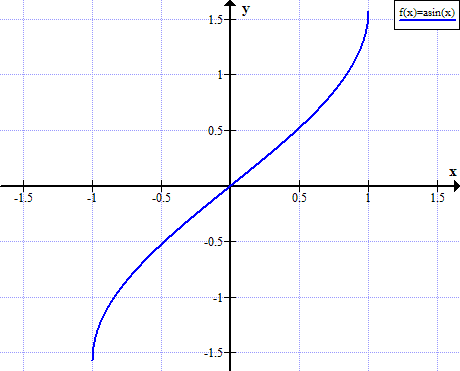### Arcsin rules

Rule name Rule
Sine of arcsine sin( arcsin x ) = x
Arcsine of sine arcsin( sin x ) = x+2kπ, when k∈ℤ (k is integer)
Arcsin of negative argument arcsin(-x) = - arcsin x
Complementary angles arcsin x = π/2 - arccos x = 90° - arccos x
Arcsin sum arcsin α + arcsin(β) = arcsin( α√(1-β2) + β√(1-α2) )
Arcsin difference arcsin α - arcsin(β) = arcsin( α√(1-β2) - β√(1-α2) )
Cosine of arcsine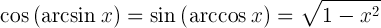Tangent of arcsine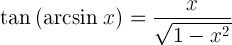Derivative of arcsine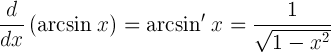Indefinite integral of arcsine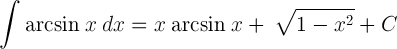x arcsin(x)

arcsin(x)

(°)

-1 -π/2 -90°
-√3/2 -π/3 -60°
-√2/2 -π/4 -45°
-1/2 -π/6 -30°
0 0
1/2 π/6 30°
2/2 π/4 45°
3/2 π/3 60°
1 π/2 90°